3228.0.55.001 - Population Estimates: Concepts, Sources and Methods, 2009
Latest ISSUE Released at 11:30 AM (CANBERRA TIME) 12/06/2009
Page tools:Print All

APPENDIX 2 EMPIRICAL BAYES ESTIMATION OF INDIGENOUS UNDERCOUNT

BACKGROUND

A2.1 Estimates of Indigenous undercount from the Census Post Enumeration Survey (PES) are required to adjust Census Indigenous figures as a key input to Indigenous estimated resident population (ERP). These estimates of Indigenous undercount adjustment should be stable (small standard errors) whilst minimising bias.

A2.2 Empirical Bayes (EB) estimation has been used by the ABS to estimate Indigenous undercount adjustments at state/territory by capital city/balance of state level - these are used to produce Indigenous ERP at state/territory level. These estimates and standard errors, prorated to ensure consistency with the Australian PES estimate, were directly produced from the EB estimator using Morris' algorithm (Morris 1983).

WHY THE EMPIRICAL BAYES APPROACH?

A2.3 High standard errors on the preliminary state/territory indigenous undercount rates lead to high sampling error for preliminary state/territory Indigenous ERP. The use of EB estimation in final rebasing in effect smoothed those parts of states with a high standard error, resulting in a more reliable undercount adjustment factor and final state/territory Indigenous ERP.

A MODEL FOR VARIATION OF UNDERCOUNT

A2.4 In estimating Indigenous numbers, the key item to be estimated from the PES is the "undercount adjustment", defined as the percentage increase to be applied to the Census count of Indigenous (after imputing for "not stated" Indigenous) to obtain a final Indigenous count. Suppose that the PES survey provides estimates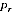with variance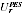of undercount adjustment for each region r, where r indexes the 15 state/territory by capital city/balance of state regions. This provides information about the distribution of the true undercount adjustmentsas follows.

A2.1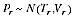where this is read: "is distributed as a normal random variable with mean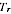and variance".

A2.5 This information is to be weighed up against a model for the likely actual variation between the true values. The information provided by this model is summarised as follows.

A2.2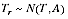A2.6 This model says that, in the absence of survey information about individual regions, we would assume that the regions had similar values. The constant A determines how different regions are likely to be in their undercount adjustments.

A2.7 A model like equation A2.2 was in fact the basis for the practice in 2001 of assuming that a single undercount adjustment should be applied to all regions. This was done in the light of the large survey error associated with PES estimates of Indigenous at state/territory level in 2001.

A2.8 Assuming first that the values A and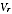are known constants, andare provided from the PES survey. The best estimate of T given equations A2.1 and A2.2 is given by:

A2.3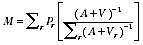and the estimate ofis:

A2.4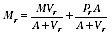A2.9 This gives a very logical outcome: where varianceis high, the value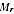is very like the overall value M, while for a region with variancelow the valueis close to the region's PES estimate.

EMPIRICAL BAYES AND THE MORRIS ALGORITHM

A2.10 In the Empirical Bayes approach the survey estimates themselves are used to estimate the variability between the underlying true values i.e. to estimate the constant A. The Morris algorithm gives a simple approach to this which should give a nearly optimal choice of A.

A2.11 First, note that under the model, with A known,

A2.5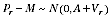A2.12 Given this, set up the random variable

A2.6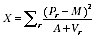A2.13 This will have a chi-squared distribution with 14 degrees of freedom (there are 15 regions, but one degree of freedom is lost by substituting the estimator M for the true value T). The expected value of X for this correct value of A is then 14.

A2.14 The Morris algorithm proceeds to find the value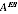which when substituted for A gives X = 14. A simple iterative algorithm achieves this. This value is then used in producing estimates from equations A2.3 and A2.4.

STABLE VARIANCE PARAMETERS

A2.15 A first issue in applying the EB methodology is that the PES survey estimates of the varianceare quite unstable, being based on the same small sample of the Indigenous population as the PES estimates themselves. Rather than use these directly, the sample sizes in each region were used in apportioning each region a share of the overall variance.

A2.16 Suppose that the PES provided a simple random sample of sizefrom the population (Indigenous and non-Indigenous), with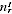turning out to be Indigenous, of whom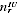were undercounted. Writing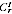for the Indigenous Census count,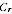for the whole Census count and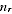for the sample size, we have expected Indigenous sample size of E() =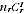/. Let the expected proportion undercounted be a constant

E(/) =.

A2.17 The PES estimate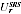of undercounted Indigenous persons in region r would then be:

A2.7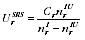A2.18 Assuming that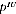is small, we have:

A2.8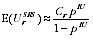and:

A2.9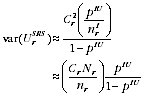A2.19 In practice PES is not a simple random sample, nor is its estimatoras simple as that above. The above development is used to justify distributing the overall PES variance across Australia in proportion to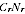/. Thus:

A2.10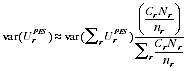A2.20 Writing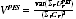for the variance of the undercount adjustment at the Australia level, as estimated directly from the PES. Noting that=/, the valueused in EB estimation is:

A2.11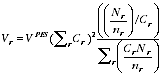A2.21 Note that the resulting parametersdo not depend on the observed sample of the Indigenous population in PES except via the overall variance estimate var(Sr).

ADJUSTING TO ADD TO THE AUSTRALIAN PES ESTIMATE

A2.22 The standard EB estimates are not guaranteed to add to the PES Indigenous estimate at Australia level. To enforce additivity to this PES estimate, a constant c was added to the undercount adjustment rates in all regions. This gave the final estimates

A2.12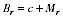A2.23 Setting the constraint:

A2.13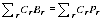and writing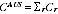and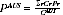gives the value of c as:

A2.14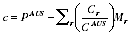THE EB ESTIMATE AS A WEIGHTED AVERAGE OF PES REGION ESTIMATES

A2.24 Using an additive adjustment as given above to ensure additivity allows the EB estimates to be written as a simple weighted sum of the region PES estimates.

A2.15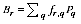where:

A2.16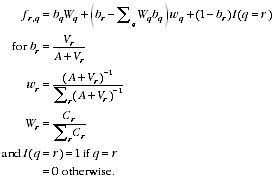VARIANCE OF EB ESTIMATE CONDITIONAL ON A

A2.25 This and the next two sections give information about the reliability of the estimates conditional on a known value of A. The effect of using the EB estimate of A is discussed in a later section.

A2.26 Since the PES estimates for each region are almost independent, the variance of the empirical Bayes estimates follows from the linear form equation A2.15 as follows:

A2.17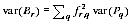A2.27 The variance estimates var(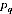) are provided by the PES estimation system based upon the observed data. They do not depend on the variance model that gave the valuesand are unbiased estimates of variance of the estimator (conditional on A) whether or not the model given by equations A2.1 and A2.2 holds.

A2.28 Note also that state estimates can be written as a weighted sum of the component region estimates, and hence as a weighted sum similar to equation A2.15. The variance of a state estimate can thus be written in a form similar to equation A2.17.

EXPECTED BIAS UNDER THE MODEL

A2.29 Since the PES estimates are design-unbiased we have E() =, and hence:

A2.18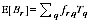A2.30 Clearly if the true valuesare treated as fixed unknown values with no underlying model, then the estimate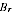is biased to the extent that the particular region r is different to other regions. So for a region with a high value ofthe estimatewill tend to be biased downwards. However, for any actual region we do not know the value of; we only observe the PES estimate. A high value ofcould be becauseis high, or because the sampling error was positive, or a combination of these. The estimatetries to balance these possibilities based on the model.

A2.31 The estimatoris unbiased forin the sense of expectation across repeated drawings from the model. Thus if we were able to repeatedly draw sets of 15 regions from the model (equation A2.2) and then get PES estimates from them with variance structure given by equation A2.1, and use them to produce estimates, then on average the bias would be zero. This is not very helpful, as even the overall mean estimate M given by equation A2.3 is unbiased in this sense.

A2.32 More useful is to measure the mean squared bias (MSB) of the estimator (or its square root, the root mean squared bias or RMSB). The MSB is zero for the PES estimate, and A for the mean estimate M. Writing EM for expectation across the model, the MSB ofis obtained as follows:

A2.19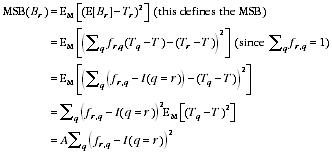ESTIMATES OF MEAN SQUARED ERROR

A2.33 Adding the MSB (equation A2.19) to the variance (equation A2.17) gives the expected mean squared error (MSE) of an EB estimate. The MSE serves as a summary of the likely size of errors from using the EB estimator. Estimates of the root MSB (RMSB) and root MSE (RMSE) are presented in the following table, alongside SE of the PES and EB estimators.

 A2.20 Estimates of SE, RMSB and RMSE for PES and EB estimates of undercount adjustment rate , States and territoriesPES EBSE RMSB RMSE SE(a) RMSB RMSE New South Wales 6.3 - 6.3 3.9 2.3 4.5 Victoria 9.9 - 9.9 3.1 4.1 5.1 Queensland 4.5 - 4.5 3.2 2.2 3.8 South Australia 10.0 - 10.0 3.3 3.9 5.1 Western Australia 8.8 - 8.8 4.2 2.9 5.1 Tasmania 7.8 - 7.8 2.9 3.9 4.9 Northern Territory 4.2 - 4.2 3.1 1.9 3.7 Australian Capital Territory 12.3 - 12.3 2.9 6.1 6.7 Australia 2.8 - 2.8 2.8 - 2.8 - nil or rounded to zero (including null cells) (a) The SE conditional on the EB value of A.

A2.34 For a hypothetical region with no PES information at all, the RMSB would be sqrt(A) = 6.6% and the RMSE would be 7.2% (larger because it still gets variance from the PES Australian estimate).

EFFECT OF ESTIMATING THE SMOOTHING CONSTANT A

A2.35 The estimatorcan be defined for any specified value of the ratio (A /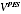), and the resulting SEs can be predicted. These SEs do not depend on the model being correct at all (though the model is required for analysis of the bias). Thus (A /) could have been chosen to give estimates with a specified size of predicted SEs. The Morris algorithm could still be used to estimatefor presentation of RMSB etc.

A2.36 In 2006, the ABS has chosen to use the estimated valuein defining the estimator. Different estimatescould have arisen, giving different estimates. Thus estimatinginduces additional variability in the estimates.

A2.37 An example can make this clear. Suppose that a very unusual estimate arises by chance. This will increase the estimated value, which in turn will lead to the estimates being smoothed less than they should be. Thus using the estimatedmakes the estimates more subject to influence of unusual estimates i.e. more variable.

A2.38 In practice, the ABS is committed to presenting stable Indigenous ERP. In the future this may lead to not using the estimated valueif it would lead to unstable estimates, or conversely an unnecessarily extreme smoothing.

A2.39 In the light of this, the ABS is content to present the SEs conditional on the chosen value. Experimental estimates show that the unconditional SE of a "pure" EB estimate which always uses the estimatedis somewhat increased over the SEs presented above. Even accounting for this, the unconditional RMSE will still be markedly lower than the SE of the PES estimates.

ALTERNATIVE MODELS AND ESTIMATORS

A2.40 It should be acknowledged that there are many alternative models that could have been used as the basis of an estimator, and alternative methods of producing the estimate. In the process of deciding to use Empirical Bayes techniques, a number of alternatives were investigated. These included modelling different classes of region (e.g. capital cities) separately, and looking for explanatory variables that could explain region differences. Different components of the undercount (e.g. the effect of misclassification as to whether a person is Indigenous) were also examined to see if predicting them separately could improve the estimator. The fit of these more sophisticated models was not sufficiently improved to justify choosing them over the simpler model (equation A2.2) that was used.

Back to top of the page